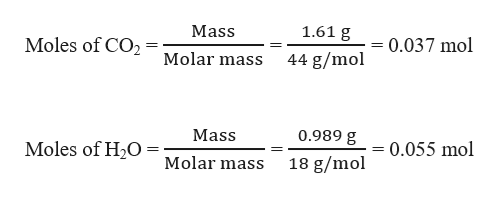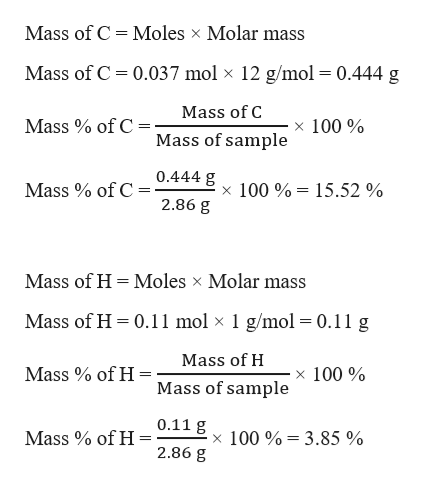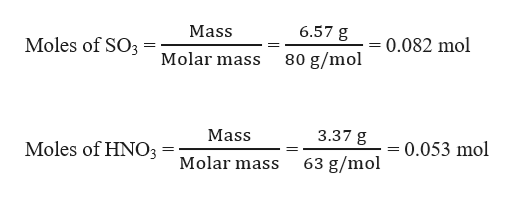# A 2.86 g sample of a compound consisting of carbon, hydrogen, oxygen, nitrogen, and sulfur was combusted in excess oxygen. This produced 1.61 g CO2 and 0.989 g H2OH2O. A second sample of this compound with a mass of 6.41 g produced 6.57 g SO3. A third sample of this compound with a mass of 8.36 g produced 3.37 g HNO3. Determine the empirical formula of the compound. Enter the correct subscripts on the given chemical formula.CHNSO

Question
346 views

A 2.86 g sample of a compound consisting of carbon, hydrogen, oxygen, nitrogen, and sulfur was combusted in excess oxygen. This produced 1.61 g CO2 and 0.989 g H2OH2O. A second sample of this compound with a mass of 6.41 g produced 6.57 g SO3. A third sample of this compound with a mass of 8.36 g produced 3.37 g HNO3. Determine the empirical formula of the compound. Enter the correct subscripts on the given chemical formula.

CHNSO

check_circle

Step 1

Given, mass of 2.86 g sample of a compound produced 1.61 g CO2 and 0.989 g H2O.

Moles of CO2 and H2O can be calculated as:help_outlineImage TranscriptioncloseMass 1.61 g Moles of CO2 0.037 mol Molar mass 44 g/mol Mass 0.989 g Moles of H20 0.055 mol Molar mass 18 g/mol fullscreen
Step 2

1 mol of CO2 contains 1 mol of C, therefore 0.037 mol of CO2 contains 0.037 mol of C.

1 mol of H2O contains 2 mol of H, therefore 0.055 mol of H2O contains (2×0.055 mol) = 0.11 mol of H.

Mass percentage of C and H in 2.86 g of sample can be calculated as:help_outlineImage TranscriptioncloseMass of C Moles x Molar mass Mass of C 0.037 mol x 12 g/mol = 0.444 g Mass of C Mass of C x 100% Mass of sample 0.444 g Mass of C x 100%15.52 % 2.86 g Mass of H Moles x Molar mass Mass of H 0.11 mol x 1 g/mol = 0.11 g Mass of H x 100% Mass % of H = Mass of sample 0.11 g Mass of H x 100% 3.85 % 2.86 g fullscreen
Step 3

Given, a second sample of this compound with a mass of 6.41 g produced 6.57 g SO3. A third sample of this compound with a mass o...help_outlineImage Transcriptionclose6.57 g Mass Moles of SO3Molar mass 0.082 mol 80 g/mol 3.37 g Mass 0.053 mol Moles of HNO3 63 g/mol Molar mass fullscreen

### Want to see the full answer?

See Solution

#### Want to see this answer and more?

Solutions are written by subject experts who are available 24/7. Questions are typically answered within 1 hour.*

See Solution
*Response times may vary by subject and question.
Tagged in

### General Chemistry